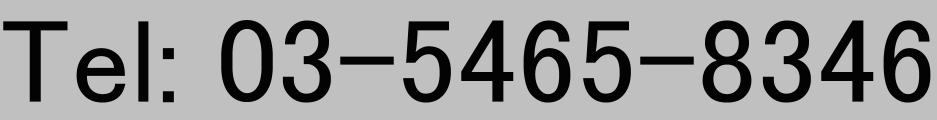# Atsushi MATSUO

Associate Professor,
Department of Mathematical Sciences
The University of Tokyo
Komaba 3-8-1, Meguro-ku, Tokyo 153-8914, Japan## Research Interests

I have been working on mathematical aspects of 2D conformal field theories and related areas.
The research topics from new to old are roughly:

• Universal formulas of minimal nilpotent orbits.
• Finiteness properties of vertex operator algebras.
• 3-transposition automorphisms of vertex operator algebras
• Vertex operator algebras with large symmetries.
• Automophism groups of vertex operator algebras.
• Representation theory of quantum affine algebras.
• Knizhnik-Zamolodchikov equation and its variants.

## Publication

### Refereed Research Papers

1. A. Matsuo and A.P. Veselov: Universal formula for the Hilbert series of minimal nilpotent orbits.
Proceedings of the American Mathematical Society., 145, No.12, (2017), 5123--5130.
2. H. Maruoka, A. Matsuo and H. Shimakura: Classification of vertex operator algebras of class S4 with minimal conformal weight one.
Journal of Mathematical Society of Japan, 68, (2016), 1369--1388.
3. A Matsuo, K. Nagatomo and A. Tsuchiya: Quasi-finite algebras graded by Hamiltonian and vertex operator algebras.
Moonshine - The First Quarter Century and Beyond, Proceedings of a Workshop on the Moonshine Conjectures and Vertex Algebras, London Mathematical Society Lecture Note Series No.\ 372, Cambridge University Press, 2010.
4. A. Matsuo: 3-transposition groups of symplectic type and vertex operator algebras.
Journal of Mathematical Society of Japan 57 (2005), no. 3, 639--649.
5. A Matsuo: Norton's trace formulae for the Griess algebra of a vertex operator algebra with larger symmetry.
Commun. Math. Phys. 224 (2001), 565--591.
6. A Matsuo and M Matsuo: The automorphism group of the Hamming code vertex operator algebra.
J. Algebra, 228 (2000), 204--226.
7. A Matsuo and K Nagatomo: A note on free bosonic vertex algebra and its conformal vectors.
J. Algebra 212 (1999), 395--418.
8. A Matsuo: A q-deformation of Wakimoto modules, primary fields, and screening operators.
Commun. Math. Phys. 160 (1994), 33--48.
9. A Matsuo: Quantum algebra structure of certain Jackson integrals.
Commun. Math. Phys., 157 (1993), 479--498.
10. A Matsuo: Free field representation of the quantum affine algebra Uq(sl^2).
Phys. Lett. B308 (1993), 260-265.
11. A Matsuo: Jackson integrals of Jordan-Pochhammer type and Quantum Knizhnik-Zamolodchikov equations.
Commun. Math. Phys., 151 (1993), 263--273.
12. A Matsuo: Integrable connections related to zonal spherical functions,
Invent. Math., 110 (1992), 95--121.
13. A Matsuo: An Application of Aomoto-Gelfand Hypergeometric Functions to SU(n) Knizhnik-Zamolodchikov Equation.
Commun. Math. Phys. 134 (1990), 65--77
14. E Date, M Jimbo, A Matsuo and T Miwa: Hypergeometric type integrals and the sl(2,C) Knizhnik-Zamolodchikov equations.
Int. J. Mod. Phys. B4 (1990), 1049

### Books

1. A Matsuo and K Nagatomo: Axioms for a vertex algebra and the locality of quantum fields.
MSJ Memoirs 4, Math. Soc. Japan, 1999.

### Other Papers

1. A Matsuo: Summary of the theory of primitive forms.
Topological field theory, primitive forms, and related topics, Birkhauser, 1998.

omitted

mail at ms